UPSC  >  Introduction & Examples (with Solutions): Pie Chart

# Introduction & Examples (with Solutions): Pie Chart - Notes | Study CSAT Preparation for UPSC CSE - UPSC

 Table of contentsWhat are Pie Charts?Some Solved Examples1 Crore+ students have signed up on EduRev. Have you?

What are Pie Charts?

Pie Charts are the circular way of data representation. In these graphs, a circle is divided into various segments and each single segment denote a category from the given data.

• In a pie chart, the arc length of each slice (and consequently its central angle and area), is proportional to the quantity it represents. Pie graphs use percentage or angles to show relative sizes of various categories. Pie charts can be used in the following ways.
• They are a visual way of displaying data that might otherwise be given in a small table.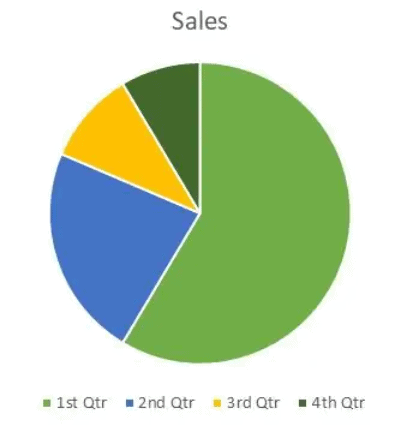• Pie charts are useful for displaying data that are classified into nominal or ordinal categories. Nominal data are categorized according to descriptive or qualitative information such as county of birth or type of pet owned. Ordinal data are similar but the different categories can also be ranked, for example in a survey people may be asked to say whether they classed something as very poor, poor, fair, good, very good.
• They are generally used to show percentage or proportional data and usually, the percentage represented by each category is provided next to the corresponding slice of pie.
• They are good for displaying data for around 6 categories or fewer. When there are more categories it is difficult for the eye to distinguish between the relative sizes of the different sectors and so the chart becomes difficult to interpret.

There are various kinds of pie graphs namely, 3D pie chart, doughnut chart, exploded pie chart, ring chart, etc. But in the exam, we will usually be given the basic pie chart which is given below.

Example:  The chart below shows the distribution of the sales of the car industry between six car companies. Looking at the chart below, we can infer that Maruti accounts for 24 percent of the market share, while GM accounts for 35 percent of the market share, Ford for4 percent of the market share, Tata for 10 percent of the market share, Hyundai for 15 percent of the market share and Fiat for 12 percent of the market share.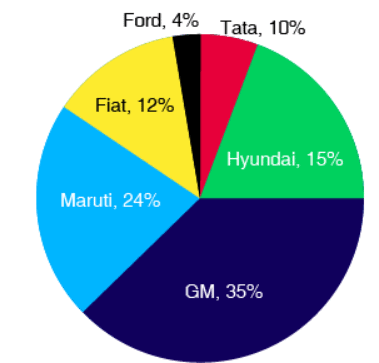Distribution of cars sales between six companies

• The pie chart encompasses a circle of 360 degrees which represents 100 percent of the value of the continuous variable. Thus, 3.6 degrees on the pie chart represent 1 percent of the total value of the continuous variable being represented.
• A single pie diagram can represent only one continuous variable. Hence, in terms of versatility of data representation, pie charts are less versatile than either bar charts, x-y graphs, or tables.

Use of a Pie Chart

1. In case you want the audience to have an understanding of the parts concerning the whole unit of data.
2. To convey a segment of data and its size.
3. It is very helpful in the presentation of data in a systematic manner to be understandable by common people as well.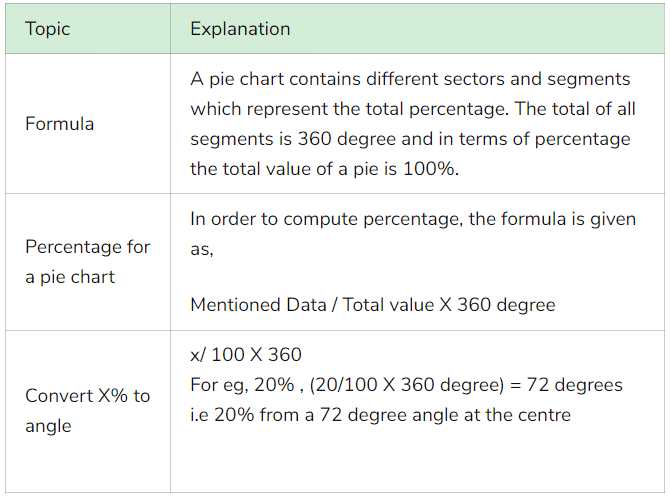Some Solved Examples

Example 1: The following pie chart shows the amount of subscriptions generated for Indian Bonds from different categories of Investors.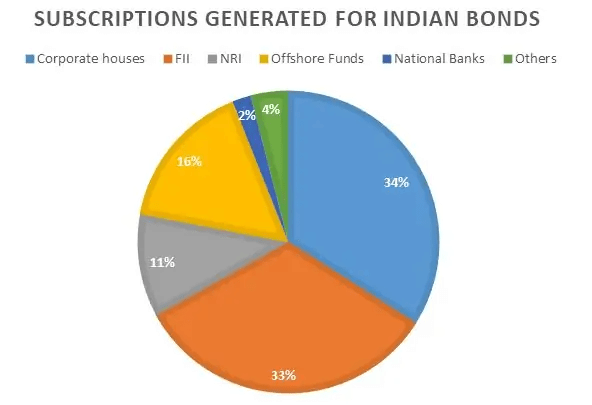(i) If the investment by NRI’s are INR 4,000 crore, then investment by both corporate houses and FII’s together is:
(a) 24000 crores
(b) 24363 crores
(c) 25423.4 crores
(d) 25643.3 crores

Solution. Option (b)
Explanation. If the investment by NRI is worth 4000 crores which are 11% of the total value.
Then, the total value will be = 4000 * 100/11 = 36363.63
Now, investment by corporate houses and FII’s together = 67% of 36363.63 = 24,363.63 crore.

(ii) What percentage of total investment is coming from either FII’s or NRI’s?
(a)
33%
(b) 44%
(c) 11%
(d) 22%
Solution. Option (b)
Explanation. Investment from FII = 33% and Invest from NRI = 11%
Thus, total investment coming from FII's or NRI's = 44%

Question for Introduction & Examples (with Solutions): Pie Chart
Try yourself:If the total investment other than by FII and corporate houses is INR 335,000 crore, then the investment by NRI’s and offshore funds will be (approximately).

(iii) What is the approximate ratio of investment flows into India Bonds from NRI’s to corporate houses?

(a) 1:4

(b) 1:3

(c) 3:1

(d) Cannot be determined

Solution: Option (b)

Explanation: The investment by NRI’s is 11% and the corporate house is 34%.
Therefore, the ratio will be 11:34 ≈ 1:3.

Question for Introduction & Examples (with Solutions): Pie Chart
Try yourself:In corporate sector, approximately how many degrees should be there in the central angle?

(iv) If the total investment flows from FII were to be doubled in the next year and the investment flows from all other sources had remained constant at their existing levels for this year, then what would be the proportion of FII investment in the total investment flows into Indian Bonds in the next year (approximately)?

(a) 40%

(b) 50%

(c) 60%

(d) 70%

Solution: Option (b)

Explanation: The current FII’s are 33% and it will double next year making it 66%.
Thus, keeping other investments constant now the total becomes 133.
Hence, 66 % of 133 will be 49.62% i.e. 50%.

Question for Introduction & Examples (with Solutions): Pie Chart
Try yourself:If the flow from the FII’s after the doubling (of previous question) were to the tune of US\$ 500 million, what would be the total investment into Indian Bond in US \$’s?

Example 2: Study the following diagram carefully and answer the questions:

Percentage Composition of Human Body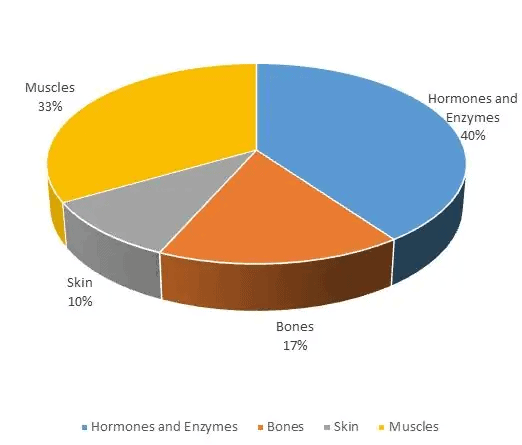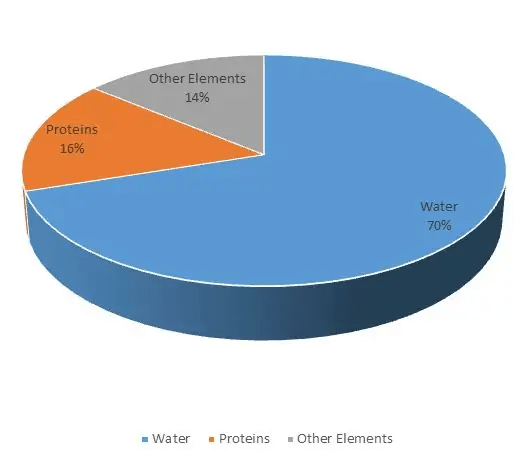(i) What percent of the total weight of human body is equivalent to the weight of the proteins in skin in human body?

(a) 0.016

(b) 1.6

(c) 0.16

(d) Cannot be determined

Solution: Now to solve this question you have to make use of both the pie charts. Since total percentage of protein in body is 16%. And, the total percentage of the weight of skin in human body is 10%. Therefore, the percentage of protein in skin is 10% of 16.i.e. 1.6.

(ii) What will be the quantity of water in the body of a person weighing 50 kg?

(a) 20 kg

(b) 35 kg

(c) 41 kg

(d) 5 kg

Solution: Water constitutes 70% of the body. Thus, if the man weighs 50 kg then 70% of it will be water. The weight of water will be 35 kgs.

(iii) What is the ratio of the distribution of proteins in the muscles to that of the distribution of proteins in the bones?

(a) 1:18

(b) 1:2

(c) 2:1

(d) 18:1

Solution:

Again, using both pie charts we know that 33% of body weight is constituted in muscles whereas 17% in bones and 16% of it will be protein.

Hence, 0.16*33: 0.16*17, which is approximately equal to 2:1.

(iv) To show the distribution of proteins and the other dry elements in the human body, the arc of the circle should subtend at the center an angle of;

(a) 54o

(b) 126o

(c) 108o

(d) 252o

Solution:

Using the formula given in the example 1, Ans 5 we get,

[(16% + 14%)/100] * 360o = 108o.

(v)  In the human body, what part is made of neither bones nor skin?

(a) 1/40

(b) 3/80

(c)  2/5

(d)  None of these

Solution:

Now let us consider the body to be- numeraire as 1 and 17/100 and 10/100 constitutes bones and skin respectively i.e. 1/6 and 1/10. Hence, the required answer is 1 – (1/6 +1/10) = 11/15.
The document Introduction & Examples (with Solutions): Pie Chart - Notes | Study CSAT Preparation for UPSC CSE - UPSC is a part of the UPSC Course CSAT Preparation for UPSC CSE.
All you need of UPSC at this link: UPSC

## CSAT Preparation for UPSC CSE

72 videos|64 docs|92 tests
 Use Code STAYHOME200 and get INR 200 additional OFF

## CSAT Preparation for UPSC CSE

72 videos|64 docs|92 tests

### How to Prepare for UPSC

Read our guide to prepare for UPSC which is created by Toppers & the best Teachers

Track your progress, build streaks, highlight & save important lessons and more!

,

,

,

,

,

,

,

,

,

,

,

,

,

,

,

,

,

,

,

,

,

;